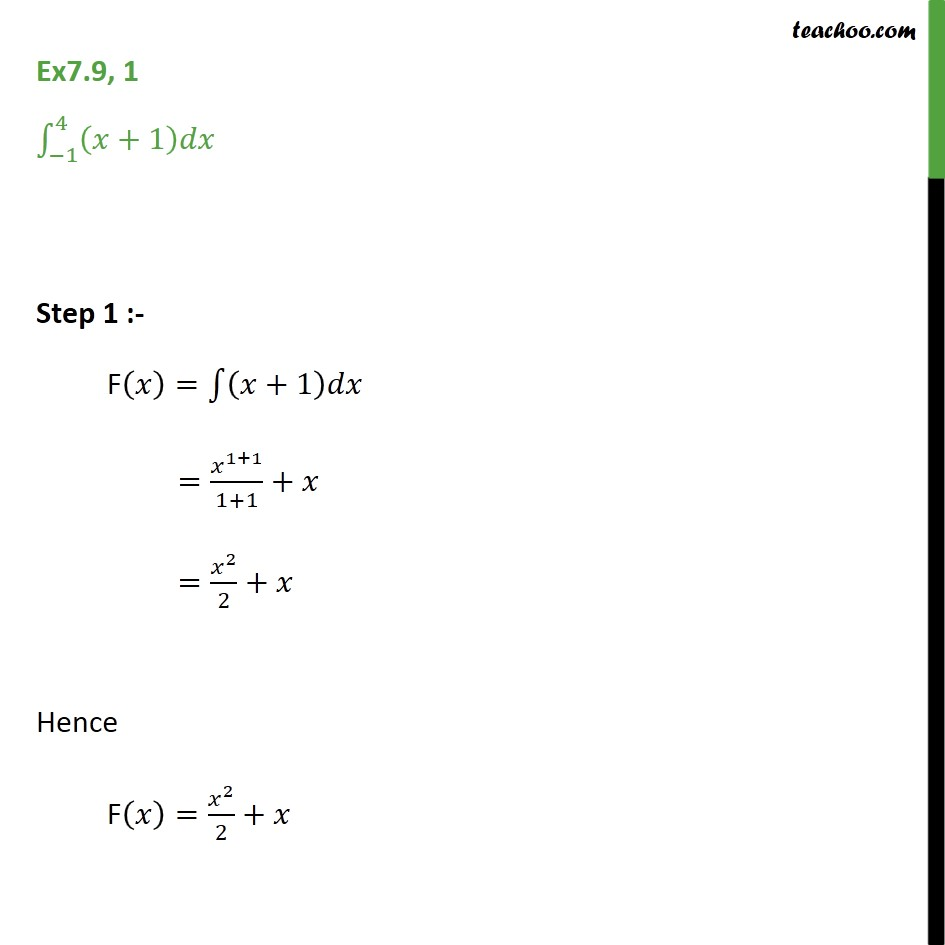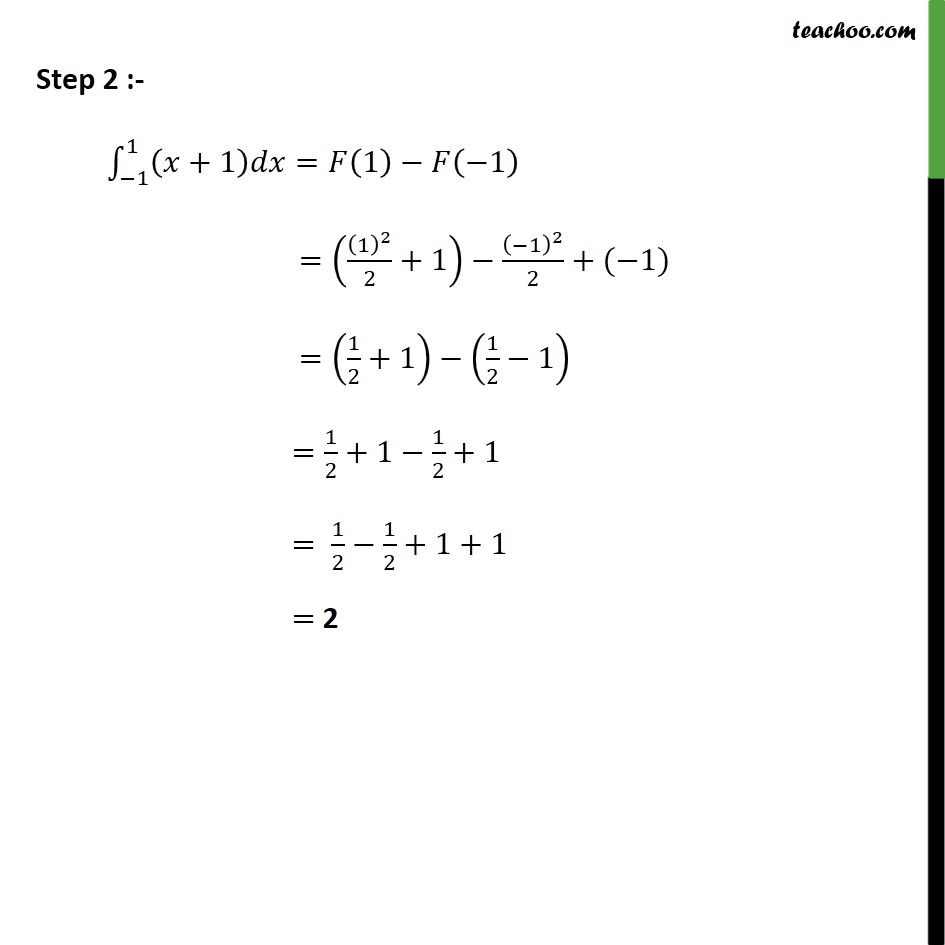Integration Full Chapter Explained - Integration Class 12 - Everything you need1. Chapter 7 Class 12 Integrals
2. Serial order wise
3. Ex 7.9

Transcript

Ex7.9, 1 1 4 +1 Step 1 :- F = +1 = 1+1 1+1 + = 2 2 + Hence F = 2 2 + Step 2 :- 1 1 +1 = 1 1 = 1 2 2 +1 1 2 2 +( 1) = 1 2 +1 1 2 1 = 1 2 +1 1 2 +1 = 1 2 1 2 +1+1 = 2

Ex 7.9

Chapter 7 Class 12 Integrals
Serial order wise